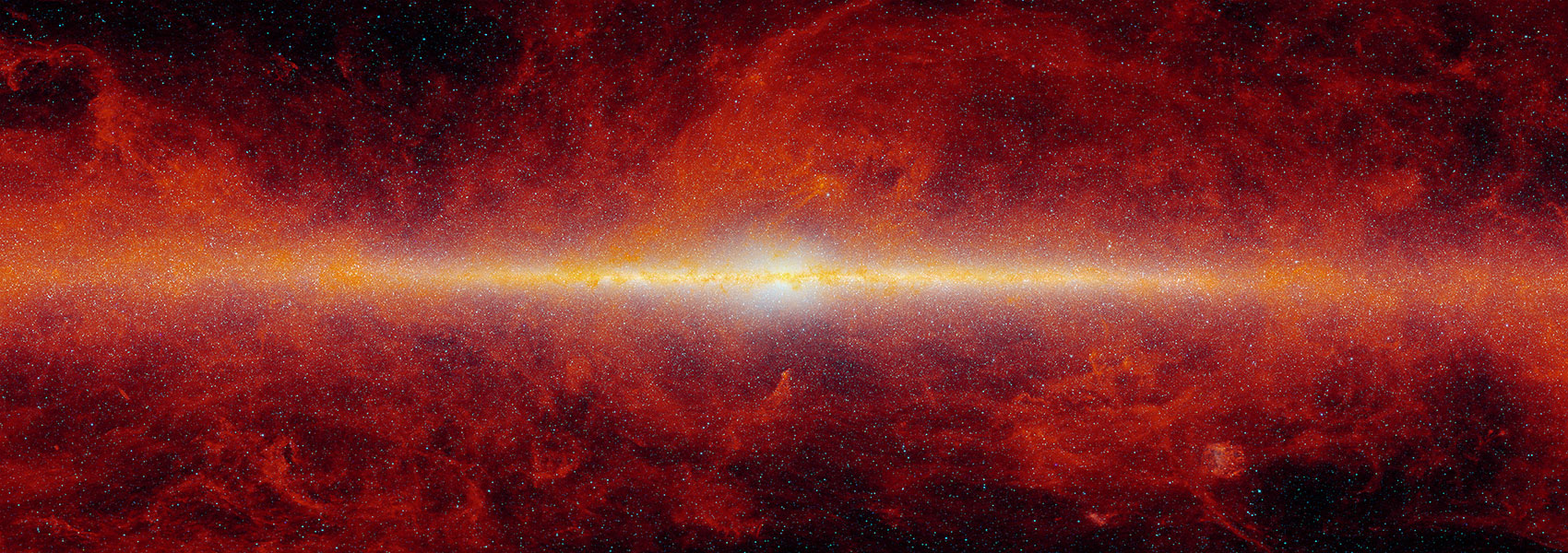# Using multipoles of the correlation function to measure H(z), DA(z) and β(z) from Sloan Digital Sky Survey luminous red galaxies

May 2013 • 2013MNRAS.431.2634C

Authors • Chuang, Chia-Hsun • Wang, Yun

Abstract • Galaxy clustering data can be used to measure the cosmic expansion history H(z), the angular diameter distance DA(z) and the linear redshift-space distortion parameter β(z). Here we present a method for using effective multipoles of the galaxy two-point correlation function (hat{ξ }_0(s), hat{ξ }_2(s), hat{ξ }_4(s) and hat{ξ }_6(s), with s denoting the comoving separation) to measure H(z), DA(z) and β(z), and validate it using LasDamas mock galaxy catalogues. Our definition of effective multipoles explicitly incorporates the discreteness of measurements, and treats the measured correlation function and its theoretical model on the same footing. We find that for the mock data, hat{ξ }_0+hat{ξ }_2+hat{ξ }_4 captures nearly all the information, and gives significantly stronger constraints on H(z), DA(z) and β(z), compared to using only hat{ξ }_0+hat{ξ }_2.

We apply our method to the sample of luminous red galaxies from the Sloan Digital Sky Survey Data Release 7 without assuming a dark energy model or a flat universe. We find that hat{ξ }_4(s) deviates on scales of s < 60 Mpc h-1 from the measurement from mock data [in contrast to hat{ξ }_0(s), hat{ξ }_2(s) and hat{ξ }_6(s)]; thus, we only use hat{ξ }_0+hat{ξ }_2 for our fiducial constraints. We obtain {H(0.35), DA(0.35), Ωmh2, β(z)} = {79.6{^{+ 8.3}_{- 8.7}} km s- 1 Mpc- 1, 1057{^{+ 88}_{- 87}} Mpc, 0.103 ± 0.015, 0.44 ± 0.15} using hat{ξ }_0+hat{ξ }_2. We find that H(0.35) rs(zd)/c and DA(0.35)/rs(zd) [where rs(zd) is the sound horizon at the drag epoch] are more tightly constrained: {H(0.35) rs(zd)/c, DA(0.35)/rs(zd)} = {0.0437{^{+ 0.0041}_{- 0.0043}},6.48{^{+ 0.44}_{- 0.43}}} using hat{ξ }_0+hat{ξ }_2.